## What is the decibel level of the radio with intensity 10−7 watts per square inch? Use a logarithmic model to solve.

Question

What is the decibel level of the radio with intensity 10−7 watts per square inch? Use a logarithmic model to solve.

in progress 0
3 months 2021-09-02T04:34:03+00:00 1 Answers 0 views 0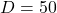Step-by-step explanation:

Given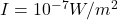— Intensity

Required

Determine the decibel level (D)

This is calculated as: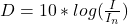Where: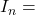The threshold intensity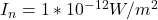So, we have:This gives: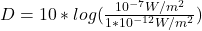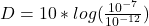Apply law of indices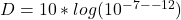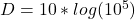Apply law of logarithm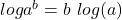So, we have: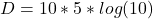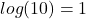So: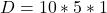Hence, the decibel level is 50